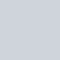# 先來看看今天的結果圖## -> 此篇文章的程式碼 github

Day06_調整色調色溫白平衡_modify_color_temperature.ipynb

# 前言

【Day4】1. 調亮光線 (調整光線)
【Day5】2. 加強飽和度 (調整飽和度)
【Day6】3. 將照片調成冷色調
4. 增添顆粒感
5. 降低對比
6. 降低高光

# 運用 OpenCV 調整色調(冷色系/暖色系)、色溫(白平衡)

``````def modify_color_temperature(img):

# ---------------- 冷色調 ---------------- #

#     height = img.shape
#     width = img.shape
#     dst = np.zeros(img.shape, img.dtype)

# 1.計算三個通道的平均值，並依照平均值調整色調
imgB = img[:, :, 0]
imgG = img[:, :, 1]
imgR = img[:, :, 2]

# 調整色調請調整這邊~~
# 白平衡 -> 三個值變化相同
# 冷色調(增加b分量) -> 除了b之外都增加
# 暖色調(增加r分量) -> 除了r之外都增加
bAve = cv2.mean(imgB)
gAve = cv2.mean(imgG) + 20
rAve = cv2.mean(imgR) + 20
aveGray = (int)(bAve + gAve + rAve) / 3

# 2. 計算各通道增益係數，並使用此係數計算結果
bCoef = aveGray / bAve
gCoef = aveGray / gAve
rCoef = aveGray / rAve
imgB = np.floor((imgB * bCoef))  # 向下取整
imgG = np.floor((imgG * gCoef))
imgR = np.floor((imgR * rCoef))

# 3. 變換後處理
#     for i in range(0, height):
#         for j in range(0, width):
#             imgb = imgB[i, j]
#             imgg = imgG[i, j]
#             imgr = imgR[i, j]
#             if imgb > 255:
#                 imgb = 255
#             if imgg > 255:
#                 imgg = 255
#             if imgr > 255:
#                 imgr = 255
#             dst[i, j] = (imgb, imgg, imgr)

# 將原文第3部分的演算法做修改版，加快速度
imgb = imgB
imgb[imgb > 255] = 255

imgg = imgG
imgg[imgg > 255] = 255

imgr = imgR
imgr[imgr > 255] = 255

cold_rgb = np.dstack((imgb, imgg, imgr)).astype(np.uint8)

print("Cold color:")
print(cold_rgb.shape)
show_img(cold_rgb)
``````

### 我們實際上做了什麼事呢?

`色溫的變化`，通常也就伴隨著「`白平衡的破壞`」。

• 想呈現「`冷酷、冰冷`」的照片，適合使用`冷色調`
• 想呈現「`溫暖、熱情`」的照片，適合使用`暖色調`

-> 關於修改演算法的部分：

# Reference

https://blog.csdn.net/weixin_39853245/article/details/101199768